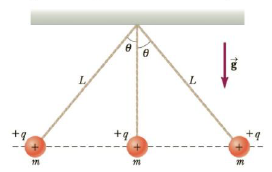Chapter 15, Problem 68AP

Chapter
Section
Textbook Problem

Three identical point charges, each of mass m = 0.100 kg, hang from three strings, as shown in Figure P15.68. If the lengths of the left and right strings are each L = 30.0 cm and if the angle θ is 45.0°, determine the value of q.Figure P15.68

To determine

The value of q.

Explanation

Given info: The mass of the charges is 0.100 kg. The length of the string is 30.0 cm.

The free body diagram is,

• FE is the net electric force.
• T is the tension.
• m is the mass of the ball.
• g is the acceleration due to gravity.

From the diagram,

Tcos45ο=mg (1)

Tsin45ο=FE (2)

Dividing the equation (2) by equation (1),

FE=mgtan45ο

Formula to calculate the net electric force is,

FE=keq2r12+keq2r22

• ke is the Coulomb constant.
• r1 and r2 are the distances of separation.
• q is the charge.

The distances between the spheres are,

r1=Lsin45οr2=2Lsin45ο

Therefore,

5keq24L2sin245ο=mgtan10ο

On Re-arranging,

q=2Lsin45οmgtan45ο5ke

Substitute 9

Still sussing out bartleby?

Check out a sample textbook solution.

See a sample solution

The Solution to Your Study Problems

Bartleby provides explanations to thousands of textbook problems written by our experts, many with advanced degrees!

Get Started

What kind of spectrum does a neon sign produce?

Horizons: Exploring the Universe (MindTap Course List)

What is genomics?

Human Heredity: Principles and Issues (MindTap Course List)

What kind of meteorite resembles Earth rocks?

Foundations of Astronomy (MindTap Course List)

How does a population differ from a community?

Oceanography: An Invitation To Marine Science, Loose-leaf Versin